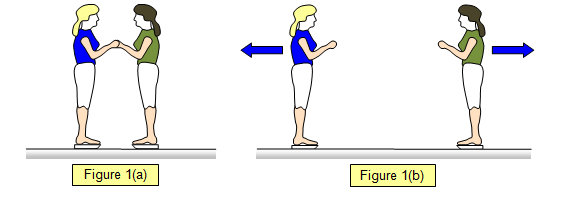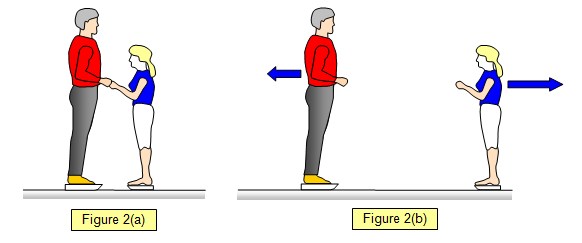# Conservation of momentum and skaters

A very good example of the conservation of momentum in an 'explosion' is given by a pair of skaters. They stand facing each other and push themselves apart, the same force acts on each skater. If their masses are equal they both recoil with the same (but opposite) velocity.Let the mass of the girl and her twin sister be m and their velocities after separation be v1 and v2.
Momentum before separation = 0
Momentum after impact = mv1 + mv2
By the law of conservation of momentum:
Momentum before they push apart = 0 = momentum after they push apart = mv1 + mv2
Therefore: 0 = mv1 + mv2 and so mv1 = - mv2 v1 = - v2

However if they are of unequal mass the person with the greater mass will move off much more slowly after the initial push.Let the mass of the girl be m, the mass of the man M and their velocities after separation be v and V.
Momentum before separation = 0
Momentum after impact = mv1 + MV
By the law of conservation of momentum:
Momentum before they push apart = 0 = momentum after they push apart = mv + MV
Therefore: 0 = mv + MV and so mv = - MV v = - [M/m]V

If the mass of the man is double that of the girl she will 'recoil' at twice his speed.### schoolphysics: Momentum conservation and skaters animation

To see an animation of skaters and the conservation of momentum click on the animation link.